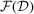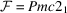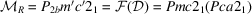International Tables for Crystallography (2016). Vol. A, ch. 3.6, pp. 852-865https://doi.org/10.1107/97809553602060000934

## Contents

• 3.6. Magnetic subperiodic groups and magnetic space groups  (pp. 852-865)
• 3.6.1. Introduction  (p. 852) | html | pdf |
• 3.6.2. Survey of magnetic subperiodic groups and magnetic space groups  (pp. 852-857) | html | pdf |
• 3.6.2.1. Reduced magnetic superfamilies of magnetic groups  (pp. 852-853) | html | pdf |
• 3.6.2.2. Survey of magnetic point groups, magnetic subperiodic groups and magnetic space groups  (pp. 853-857) | html | pdf |
• 3.6.2.2.1. Magnetic group type serial number  (p. 853) | html | pdf |
• 3.6.2.2.2. Magnetic group type symbol  (pp. 853-854) | html | pdf |
• 3.6.2.2.3. Standard set of coset representatives  (p. 854) | html | pdf |
• 3.6.2.2.4. Opechowski–Guccione magnetic group type symbols and the standard set of coset representatives  (pp. 854-856) | html | pdf |
• 3.6.2.2.5. Symbol of the subgroupof index 2 of(pp. 856-857) | html | pdf |
• 3.6.3. Tables of properties of magnetic groups  (pp. 857-863) | html | pdf |
• 3.6.3.1. Lattice diagram  (p. 857) | html | pdf |
• 3.6.3.2. Heading  (pp. 857-858) | html | pdf |
• 3.6.3.3. Diagrams of symmetry elements and of the general positions  (pp. 859-861) | html | pdf |
• 3.6.3.4. Origin  (p. 861) | html | pdf |
• 3.6.3.5. Asymmetric unit  (p. 861) | html | pdf |
• 3.6.3.6. Symmetry operations  (pp. 861-862) | html | pdf |
• 3.6.3.7. Abbreviated headline  (p. 862) | html | pdf |
• 3.6.3.8. Generators selected  (p. 862) | html | pdf |
• 3.6.3.9. General and special positions with spins (magnetic moments)  (p. 862) | html | pdf |
• 3.6.3.10. Symmetry of special projections  (pp. 862-863) | html | pdf |
• 3.6.4. Comparison of OG and BNS magnetic group type symbols  (p. 863) | html | pdf |
• 3.6.5. Maximal subgroups of index ≤ 4  (pp. 863-864) | html | pdf |
• References | html | pdf |
• Figures
• Fig. 3.6.3.1. Table of properties of the three-dimensional magnetic space group 51.13.399 P2bmma  (pp. 858-859) | html | pdf |
• Fig. 3.6.3.2. Lattice diagrams of (a) the three-dimensional magnetic space group 26.1.168and (b) the three-dimensional magnetic space group 26.10.177(p. 858) | html | pdf |
• Fig. 3.6.3.3. Symmetry diagram of P412′2′  (p. 860) | html | pdf |
• Fig. 3.6.3.4. General-position diagram of P412′2′  (p. 860) | html | pdf |
• Fig. 3.6.3.5. Diagrams of the magnetic space group P41221′  (p. 860) | html | pdf |
• Fig. 3.6.3.6. General-position diagram of rod group 2.3.29 P2/c′11  (p. 860) | html | pdf |
• Tables
• Table 3.6.2.1. Numbers of types of groups in the reduced magnetic superfamilies of one-, two- and three-dimensional crystallographic point groups, subperiodic groups and space groups  (p. 853) | html | pdf |
• Table 3.6.2.2. Examples of the format of the survey of magnetic groups of three-dimensional magnetic space-group types  (p. 853) | html | pdf |
• Table 3.6.3.1. Symmetry operations of magnetic space group 51.14.400 P2bmma  (p. 861) | html | pdf |
• Table 3.6.3.2. General positions of magnetic space group 51.14.400 P2bmma  (p. 862) | html | pdf |
• Table 3.6.4.1. Comparisons of three-dimensional OG and BNS magnetic group type symbols  (p. 863) | html | pdf |